Multiplying Fractions

Remember when multiplying fractions, multiply the numerators (tops), and then multiply the denominators (bottoms). For instance,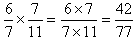. Notice that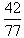can be simplified, since 7 divides evenly into the numerator and denominator,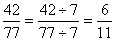. So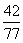is the correct answer, but can be simplified to be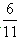.

To avoid working with larger numbers, simplify first. Notice that there is a factor of 7 in the numerator and denominator, which can be cancelled before multiplying.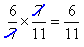Cancelling before multiplying is a great time-saver and prevents many common mistakes.

Hint: If the same factor appears in the numerator and the denominator, cancel it before multiplying. This works regardless of whether the numbers appear in the same fraction or different fractions.*Caution: This rule only applies when you are multiplying fractions; not when you are adding, subtracting, or dividing.